# Bernstein inequality

(diff) ← Older revision | Latest revision (diff) | Newer revision → (diff)
Jump to: navigation, search

Bernstein's inequality in probability theory is a more precise formulation of the classical Chebyshev inequality in probability theory, proposed by S.N. Bernshtein  in 1911; it permits one to estimate the probability of large deviations by a monotone decreasing exponential function (cf. Probability of large deviations). In fact, if the equations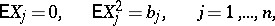hold for the independent random variableswith(where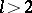and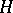is a constant independent of), then the following inequality of Bernstein (where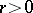) is valid for the sum: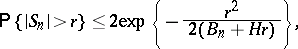(1)

where. For identically-distributed bounded random variables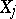(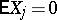,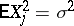and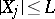,) inequality (1) takes its simplest form:(2)

where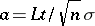. A.N. Kolmogorov gave a lower estimate of the probability in (1). The Bernstein–Kolmogorov estimates are used, in particular, in proving the law of the iterated logarithm. Some idea of the accuracy of (2) may be obtained by comparing it with the approximate value of the left-hand side of (2) which is obtained by the central limit theorem in the form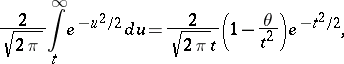where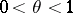. Subsequent to 1967, Bernstein's inequalities were extended to include multi-dimensional and infinite-dimensional cases.